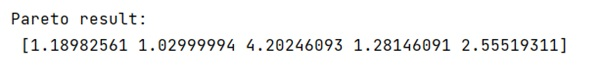# scipy.stats seed

Learn about the scipy.stats and we will understand if is there any method like scipy stats.seed just like numpy.random.seed().
Submitted by Pranit Sharma, on February 25, 2023

NumPy is an abbreviated form of Numerical Python. It is used for different types of scientific operations in python. Numpy is a vast library in python which is used for almost every kind of scientific or mathematical operation. It is itself an array which is a collection of various methods and functions for processing the arrays.

Scipy is an abbreviated form of Scientific Python. It is a scientific computation library that provides utility functions and offers more optimization and signal processing. Scipy is an open-source library that can be used freely. Scipy uses Numpy to run its added optimized functions in the field of Data Science.

The scipy.stats just uses numpy.random to generate its random numbers, so it is very similar to numpy.random.seed().

The scipy provides pareto which is a pareto continuous random variable. It is an instance of the rv_continuous class, pareto object inherits from it a collection of generic methods and completes them with details specific to this particular distribution.

Let us understand with the help of an example,

## Python code to demonstrate the scipy.stats seed

```# Import numpy
import numpy as np

# Import scipy pareto
from scipy.stats import pareto

x = 0.9

# Using scipy stats pareto
res = pareto.rvs(x, loc=0, scale=1, size=5)

# Display result
print("Pareto result:\n",res)
```

Output:Languages: » C » C++ » C++ STL » Java » Data Structure » C#.Net » Android » Kotlin » SQL
Web Technologies: » PHP » Python » JavaScript » CSS » Ajax » Node.js » Web programming/HTML
Solved programs: » C » C++ » DS » Java » C#
Aptitude que. & ans.: » C » C++ » Java » DBMS
Interview que. & ans.: » C » Embedded C » Java » SEO » HR
CS Subjects: » CS Basics » O.S. » Networks » DBMS » Embedded Systems » Cloud Computing
» Machine learning » CS Organizations » Linux » DOS
More: » Articles » Puzzles » News/Updates